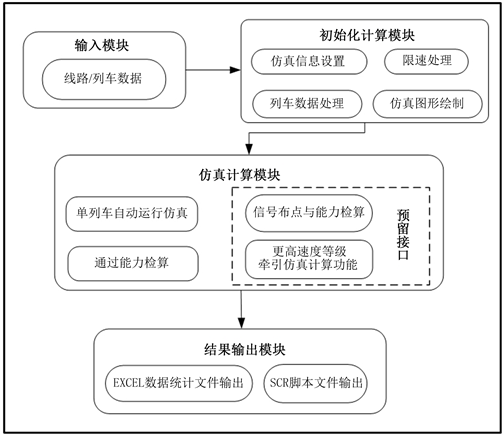﻿ 时速400 km高铁线路能力检算仿真系统研究

# 时速400 km高铁线路能力检算仿真系统研究Research on the Simulation System for Checking and Calculating the Capacity of High-Speed Railway Lines at 400 km/h

Abstract: The 400 km/h high-speed rail is still in the design stage. Through train operation simulation, the passing capacity and other technical and economic indicators under a given line and signal design plan are accurately evaluated, which helps to improve the rationality of the design plan. This paper analyzes the functional deficiencies of the existing high-speed railway train operation simulation software, and combines the requirements of high-speed railway design for train operation simulation, and designs a 400 km/h high-speed railway line signal layout capacity check simulation system. Finally, based on the existing high-speed rail lines, the simulation system is used to compare and analyze the differences in running time, traction energy consumption and passing capacity of 400 km/h electric multiple unit (EMU) and 350 km/h EMU.

1. 引言

2. 系统设计

2.1. 系统功能需求

1) 400 km/h速度等级单列车运行仿真及运行指标计算

2) 400 km/h速度等级高速铁路线路通过能力检算

2.2. 系统结构设计Figure 1. The frame structure of the system

3. 系统功能实现

3.1. 基础数据管理

3.2. 列车运行仿真功能

3.2.1. 受力分析

1) 牵引力

$\left\{\begin{array}{l}{f}_{i}={f}_{i1}+\frac{\left({f}_{i0}-{f}_{i1}\right)\cdot \left({v}_{1}-v\right)}{{v}_{1}-{v}_{0}}\\ {f}_{j}={f}_{j1}+\frac{\left({f}_{j0}-{f}_{j1}\right)\cdot \left({v}_{1}-v\right)}{{v}_{1}-{v}_{0}}\\ {f}_{k}={f}_{j}+\frac{\left({f}_{j0}-{f}_{j1}\right)\cdot \left({n}_{k}-{n}_{j}\right)}{{n}_{i}-{n}_{j}}\end{array}$ (1)

2) 阻力

${W}_{0}\left({v}_{t}\right)=a+b×{v}_{t}+c×{v}_{t}^{2}$ (2)

${W}_{j}\left(X\right)=\text{\hspace{0.17em}}{w}_{i}\left(X\right)\text{\hspace{0.17em}}+{w}_{r}\left(X\right)+{w}_{s}\left(X\right)$ (3)

$\omega \left({v}_{t},X\right)=\text{\hspace{0.17em}}\frac{\left({W}_{0}\left({v}_{t}\right)+{W}_{j}\left(X\right)\right)\cdot M\cdot g}{1000}$ (4)

3) 制动力

3.2.2. 运动方程

$\alpha =\frac{F\left({v}_{t}\right)-B\left({v}_{t}\right)-\omega \left({v}_{t},X\right)}{\left(1+\gamma \right)M}$ (5)

${v}_{t+1}={v}_{t}+\alpha \cdot \Delta t$ (6)

${S}_{t+1}={S}_{t}+\left({v}_{t}\cdot \Delta t+\frac{1}{2}\alpha \cdot \Delta {t}^{2}\right)/3.6$ (7)

3.3. 通过能力检算

3.3.1. 区间通过能力检算

${I}_{区}=3.6\frac{{L}_{区}}{{v}_{运}}=3.6\frac{{l}_{列}+{l}_{分区}+{l}_{防}+{l}_{制}}{{v}_{运}}+{t}_{附}$ (8)

3.3.2. 车站通过能力检算

1) 车站出发间隔时间

${I}_{发}=3.6\frac{{L}_{发}}{{v}_{运}}=3.6\frac{{l}_{防}+{l}_{岔区}+{l}_{离去}+{l}_{列}}{{v}_{运}}+{t}_{发作}$ (9)

2) 车站到达间隔时间

${I}_{到}=3.6\frac{{L}_{到}}{{v}_{运}}=3.6\frac{{l}_{列}+{l}_{岔区}+{l}_{防}+{l}_{制}}{{v}_{运}}+{t}_{到作}+{t}_{附}$ (10)

3) 车站通过间隔时间

${I}_{通}=3.6\frac{{l}_{列}+{l}_{站区}+{l}_{防}+{l}_{制}}{{v}_{运}}+{t}_{附}$ (11)

4. 案例分析Table 1. Index results of train operation simulationTable 2. Results of passing ability check

5. 结论

 Howlett, P. (1990) An Optimal Strategy for the Control of a Train. The Journal of the Australian Mathematical Society, 31, 454-471.
https://doi.org/10.1017/S0334270000006780

 钟世富, 曹震, 张杰. CRH动车组列车牵引计算系统研究与设计[J]. 铁路计算机应用, 2014, 23(1): 10-13.

 何桥. 高速动车组牵引计算仿真系统设计与开发[D]: [硕士学位论文]. 四川: 西南交通大学, 2013.

 毛保华, 何天键, 袁振洲, 等. 通用列车运行模拟软件系统研究[J]. 铁道学报, 2000(1): 1-6.

 丁勇, 毛保华, 刘海东, 等. 列车节能运行模拟系统的研究[J]. 北方交通大学学报, 2004, 28(2): 76-82.

 王月仙, 王成国, 马大炜, 等. 高速动车组自动运行仿真研究[J]. 电力机车与城轨车辆, 2009, 32(4): 7-10.

 何桥, 尹元钊. 动车组牵引计算仿真系统设计[J]. 交通科技与经济, 2013, 15(4): 82-84+89.

 马少坡. 动车组牵引计算仿真研究[J]. 铁道建筑技术, 2013(S1): 243-246+267.

 唐金金, 周磊山, 佟路, 等. 单列高速列车运行仿真模型与算法[J]. 中国铁道科学, 2012(3): 109-115.

 唐金金, 周磊山. 高速列车运行仿真系统研究[J]. 物流技术, 2011, 30(13): 113-115.

 吕希奎, 杨峰, 王奇胜. 高速铁路牵引计算与仿真系统研究[J]. 铁道标准设计, 2019, 63(4): 9-16.

Top